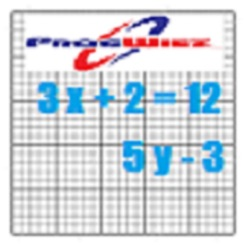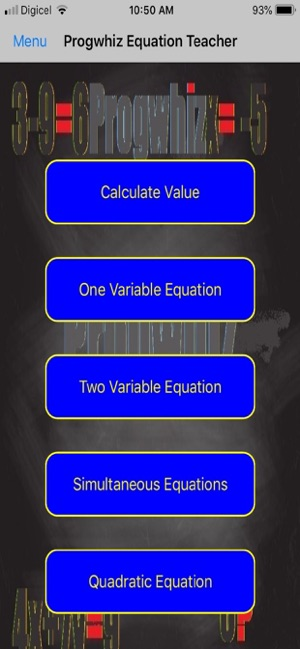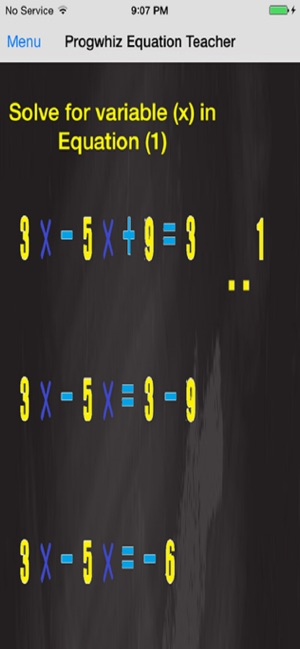## 屏幕快照

••## 简介

The Progwhiz Mathematics Teaching tool is to teach students both the fundamentals of Algebra as well as the methodology and steps to solve linear equations to find the value of unknown variables ( 1 to 2 unknowns). Such as One variable and two variable equations (Simultaneous Equations, Quadratic Equations, Factorisation).
The educational application allows the student to key in his/her equation problem and not only provide a solutions but also show all the steps in the workflow to solve the problem.

## 新内容

Improved rounding of decimals

## 信息

Mark Raymond

5.2 MB

Copyright
© Progwhiz Solutions

¥18.00

## 支持

•